# Working with Excel Spreadsheets in Python

• Last Updated : 12 May, 2021

You all must have worked with Excel at some time in your life and must have felt the need for automating some repetitive or tedious task. Don’t worry in this tutorial we are going to learn about how to work with Excel using Python, or automating Excel using Python. We will be covering this with the help of the Openpyxl module.

## Getting Started

Openpyxl is a Python library that provides various methods to interact with Excel Files using Python. It allows operations like reading, writing, arithmetic operations, plotting graphs, etc.

This module does not come in-built with Python. To install this type the below command in the terminal.

`pip install openpyxl`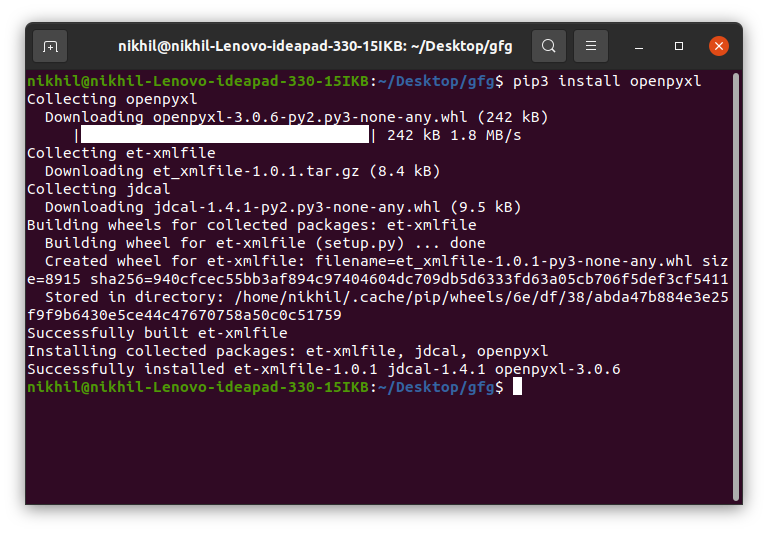To read an Excel file you have to open the spreadsheet using the load_workbook() method. After that, you can use the active to select the first sheet available and the cell attribute to select the cell by passing the row and column parameter. The value attribute prints the value of the particular cell. See the below example to get a better understanding.

Note: The first row or column integer is 1, not 0.Example:

## Python3

 `# Python program to read an excel file `` ` `# import openpyxl module ``import` `openpyxl `` ` `# Give the location of the file ``path ``=` `"gfg.xlsx"`` ` `# To open the workbook ``# workbook object is created ``wb_obj ``=` `openpyxl.load_workbook(path) `` ` `# Get workbook active sheet object ``# from the active attribute ``sheet_obj ``=` `wb_obj.active `` ` `# Cell objects also have a row, column, ``# and coordinate attributes that provide ``# location information for the cell. `` ` `# Note: The first row or ``# column integer is 1, not 0. `` ` `# Cell object is created by using ``# sheet object's cell() method. ``cell_obj ``=` `sheet_obj.cell(row ``=` `1``, column ``=` `1``) `` ` `# Print value of cell object ``# using the value attribute ``print``(cell_obj.value) `

Output:

`Name`

There can be two ways of reading from multiple cells.

Method 1: We can get the count of the total rows and columns using the max_row and max_column respectively. We can use these values inside the for loop to get the value of the desired row or column or any cell depending upon the situation. Let’s see how to get the value of the first column and first row.

Example:

## Python3

 `# Python program to read an excel file `` ` `# import openpyxl module ``import` `openpyxl `` ` `# Give the location of the file ``path ``=` `"gfg.xlsx"`` ` `# To open the workbook ``# workbook object is created ``wb_obj ``=` `openpyxl.load_workbook(path) `` ` `# Get workbook active sheet object ``# from the active attribute ``sheet_obj ``=` `wb_obj.active `` ` `# Getting the value of maximum rows``# and column``row ``=` `sheet_obj.max_row``column ``=` `sheet_obj.max_column`` ` `print``(``"Total Rows:"``, row)``print``(``"Total Columns:"``, column)`` ` `# printing the value of first column``# Loop will print all values ``# of first column  ``print``(``"\nValue of first column"``)``for` `i ``in` `range``(``1``, row ``+` `1``): ``    ``cell_obj ``=` `sheet_obj.cell(row ``=` `i, column ``=` `1``) ``    ``print``(cell_obj.value) ``     ` `# printing the value of first column``# Loop will print all values ``# of first row``print``(``"\nValue of first row"``)``for` `i ``in` `range``(``1``, column ``+` `1``): ``    ``cell_obj ``=` `sheet_obj.cell(row ``=` `2``, column ``=` `i) ``    ``print``(cell_obj.value, end ``=` `" "``)`

Output:

```Total Rows: 6
Total Columns: 4

Value of first column
Name
Ankit
Rahul
Priya
Nikhil
Nisha

Value of first row
Ankit  B.Tech CSE 4 ```

Method 2: We can also read from multiple cells using the cell name. This can be seen as the list slicing of Python.

## Python3

 `# Python program to read an excel file `` ` `# import openpyxl module ``import` `openpyxl `` ` `# Give the location of the file ``path ``=` `"gfg.xlsx"`` ` `# To open the workbook ``# workbook object is created ``wb_obj ``=` `openpyxl.load_workbook(path) `` ` `# Get workbook active sheet object ``# from the active attribute ``sheet_obj ``=` `wb_obj.active `` ` `# Cell object is created by using ``# sheet object's cell() method. ``cell_obj ``=` `sheet_obj[``'A1'``: ``'B6'``]`` ` `# Print value of cell object ``# using the value attribute ``for` `cell1, cell2 ``in` `cell_obj:``    ``print``(cell1.value, cell2.value)`

Output:

```Name Course
Ankit  B.Tech
Rahul M.Tech
Priya MBA
Nikhil B.Tech
Nisha B.Tech```

Refer to the below article to get detailed information about reading excel files using openpyxl.

First, let’s create a new spreadsheet, and then we will write some data to the newly created file. An empty spreadsheet can be created using the Workbook() method. Let’s see the below example.

Example:

## Python3

 `from` `openpyxl ``import` `Workbook`` ` `# Call a Workbook() function of openpyxl  ``# to create a new blank Workbook object ``workbook ``=` `Workbook()`` ` `# Anytime you modify the Workbook object ``# or its sheets and cells, the spreadsheet ``# file will not be saved until you call ``# the save() workbook method. ``workbook.save(filename``=``"sample.xlsx"``)`

Output: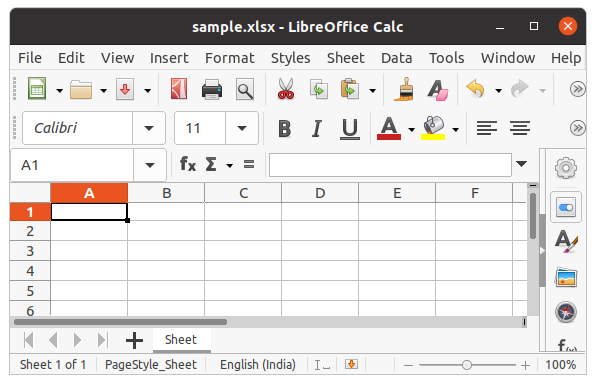After creating an empty file, let’s see how to add some data to it using Python. To add data first we need to select the active sheet and then using the cell() method we can select any particular cell by passing the row and column number as its parameter. We can also write using cell names. See the below example for a better understanding.

Example:

## Python3

 `# import openpyxl module ``import` `openpyxl `` ` `# Call a Workbook() function of openpyxl ``# to create a new blank Workbook object ``wb ``=` `openpyxl.Workbook() `` ` `# Get workbook active sheet ``# from the active attribute ``sheet ``=` `wb.active `` ` `# Cell objects also have row, column ``# and coordinate attributes that provide ``# location information for the cell. `` ` `# Note: The first row or column integer ``# is 1, not 0. Cell object is created by ``# using sheet object's cell() method. ``c1 ``=` `sheet.cell(row ``=` `1``, column ``=` `1``) `` ` `# writing values to cells ``c1.value ``=` `"Hello"`` ` `c2 ``=` `sheet.cell(row``=` `1` `, column ``=` `2``) ``c2.value ``=` `"World"`` ` `# Once have a Worksheet object, one can ``# access a cell object by its name also. ``# A2 means column = 1 & row = 2. ``c3 ``=` `sheet[``'A2'``] ``c3.value ``=` `"Welcome"`` ` `# B2 means column = 2 & row = 2. ``c4 ``=` `sheet[``'B2'``] ``c4.value ``=` `"Everyone"`` ` `# Anytime you modify the Workbook object ``# or its sheets and cells, the spreadsheet ``# file will not be saved until you call ``# the save() workbook method. ``wb.save(``"sample.xlsx"``) `

Output: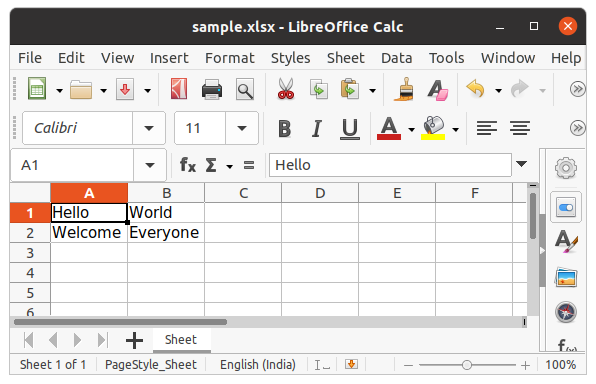Refer to the below article to get detailed information about writing to excel.

In the above example, you will see that every time you try to write to a spreadsheet the existing data gets overwritten, and the file is saved as a new file. This happens because the Workbook() method always creates a new workbook file object. To write to an existing workbook you must open the file with the load_workbook() method. We will use the above-created workbook.

Example:

## Python3

 `# import openpyxl module ``import` `openpyxl `` ` `wb ``=` `openpyxl.load_workbook(``"sample.xlsx"``) `` ` `sheet ``=` `wb.active `` ` `c ``=` `sheet[``'A3'``] ``c.value ``=` `"New Data"`` ` `wb.save(``"sample.xlsx"``)`

Output: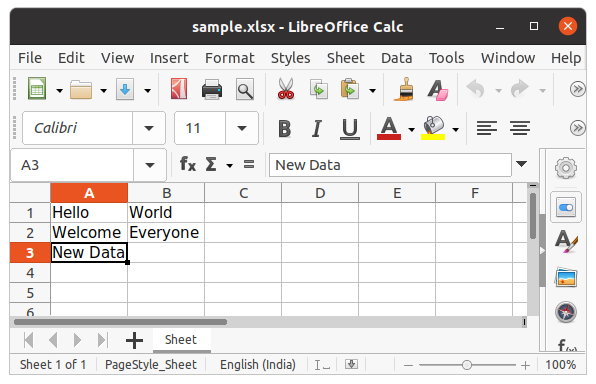We can also use the append() method to append multiple data at the end of the sheet.

Example:

## Python3

 `# import openpyxl module ``import` `openpyxl `` ` `wb ``=` `openpyxl.load_workbook(``"sample.xlsx"``) `` ` `sheet ``=` `wb.active `` ` `data ``=` `(``    ``(``1``, ``2``, ``3``),``    ``(``4``, ``5``, ``6``)``)`` ` `for` `row ``in` `data:``    ``sheet.append(row)`` ` `wb.save(``'sample.xlsx'``)`

Output: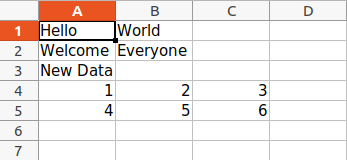Arithmetic operations can be performed by typing the formula in a particular cell of the spreadsheet. For example, if we want to find the sum then =Sum() formula of the excel file is used.

Example:

## Python3

 `# import openpyxl module ``import` `openpyxl `` ` `# Call a Workbook() function of openpyxl ``# to create a new blank Workbook object ``wb ``=` `openpyxl.Workbook() `` ` `# Get workbook active sheet ``# from the active attribute. ``sheet ``=` `wb.active `` ` `# writing to the cell of an excel sheet ``sheet[``'A1'``] ``=` `200``sheet[``'A2'``] ``=` `300``sheet[``'A3'``] ``=` `400``sheet[``'A4'``] ``=` `500``sheet[``'A5'``] ``=` `600`` ` `# The value in cell A7 is set to a formula ``# that sums the values in A1, A2, A3, A4, A5 . ``sheet[``'A7'``] ``=` `'= SUM(A1:A5)'`` ` `# save the file ``wb.save(``"sum.xlsx"``) `

Output: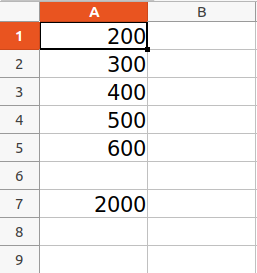Refer to the below article to get detailed information about the Arithmetic operations on Spreadsheet.

Worksheet objects have row_dimensions and column_dimensions attributes that control row heights and column widths. A sheet’s row_dimensions and column_dimensions are dictionary-like values; row_dimensions contains RowDimension objects and column_dimensions contains ColumnDimension objects. In row_dimensions, one can access one of the objects using the number of the row (in this case, 1 or 2). In column_dimensions, one can access one of the objects using the letter of the column (in this case, A or B).

Example:

## Python3

 `# import openpyxl module ``import` `openpyxl `` ` `# Call a Workbook() function of openpyxl ``# to create a new blank Workbook object ``wb ``=` `openpyxl.Workbook() `` ` `# Get workbook active sheet ``# from the active attribute. ``sheet ``=` `wb.active `` ` `# writing to the specified cell ``sheet.cell(row ``=` `1``, column ``=` `1``).value ``=` `' hello '`` ` `sheet.cell(row ``=` `2``, column ``=` `2``).value ``=` `' everyone '`` ` `# set the height of the row ``sheet.row_dimensions[``1``].height ``=` `70`` ` `# set the width of the column ``sheet.column_dimensions[``'B'``].width ``=` `20`` ` `# save the file ``wb.save(``'sample.xlsx'``) `

Output: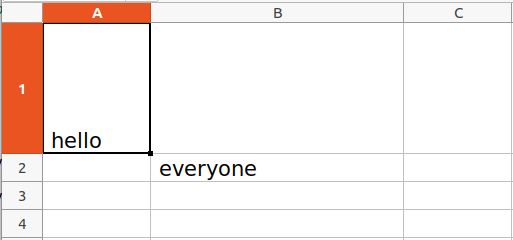### Merging Cells

A rectangular area of cells can be merged into a single cell with the merge_cells() sheet method. The argument to merge_cells() is a single string of the top-left and bottom-right cells of the rectangular area to be merged.

Example:

## Python3

 `import` `openpyxl ``wb ``=` `openpyxl.Workbook() ``sheet ``=` `wb.active `` ` `# merge cell from A2 to D4 i.e. ``# A2, B2, C2, D2, A3, B3, C3, D3, A4, B4, C4 and D4 . ``# A2:D4' merges 12 cells into a single cell. ``sheet.merge_cells(``'A2:D4'``) `` ` `sheet.cell(row ``=` `2``, column ``=` `1``).value ``=` `'Twelve cells join together.'`` ` `# merge cell C6 and D6 ``sheet.merge_cells(``'C6:D6'``) `` ` `sheet.cell(row ``=` `6``, column ``=` `6``).value ``=` `'Two merge cells.'`` ` `wb.save(``'sample.xlsx'``)`

Output: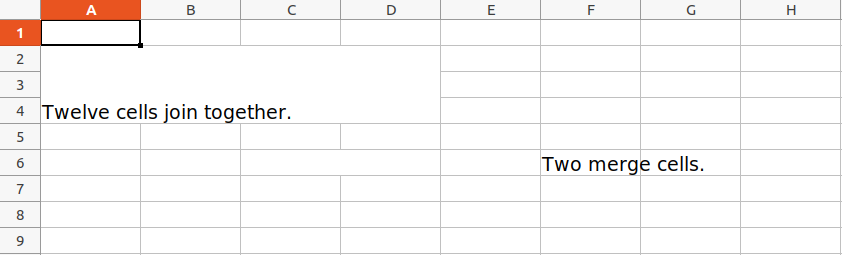### Unmerging Cells

To unmerge cells, call the unmerge_cells() sheet method.

Example:

## Python3

 `import` `openpyxl `` ` ` ` `wb ``=` `openpyxl.load_workbook(``'sample.xlsx'``) ``sheet ``=` `wb.active `` ` `# unmerge the cells ``sheet.unmerge_cells(``'A2:D4'``) `` ` `sheet.unmerge_cells(``'C6:D6'``) `` ` `wb.save(``'sample.xlsx'``)`

Output: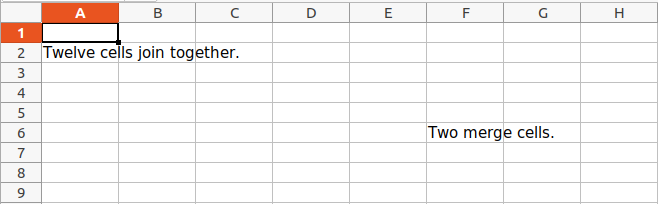### Setting Font Style

To customize font styles in cells, important, import the Font() function from the openpyxl.styles module.

Example:

## Python3

 `import` `openpyxl `` ` `# import Font function from openpyxl ``from` `openpyxl.styles ``import` `Font `` ` ` ` `wb ``=` `openpyxl.Workbook() ``sheet ``=` `wb.active `` ` `sheet.cell(row ``=` `1``, column ``=` `1``).value ``=` `"GeeksforGeeks"`` ` `# set the size of the cell to 24 ``sheet.cell(row ``=` `1``, column ``=` `1``).font ``=` `Font(size ``=` `24` `) `` ` `sheet.cell(row ``=` `2``, column ``=` `2``).value ``=` `"GeeksforGeeks"`` ` `# set the font style to italic ``sheet.cell(row ``=` `2``, column ``=` `2``).font ``=` `Font(size ``=` `24``, italic ``=` `True``) `` ` `sheet.cell(row ``=` `3``, column ``=` `3``).value ``=` `"GeeksforGeeks"`` ` `# set the font style to bold ``sheet.cell(row ``=` `3``, column ``=` `3``).font ``=` `Font(size ``=` `24``, bold ``=` `True``) `` ` `sheet.cell(row ``=` `4``, column ``=` `4``).value ``=` `"GeeksforGeeks"`` ` `# set the font name to 'Times New Roman' ``sheet.cell(row ``=` `4``, column ``=` `4``).font ``=` `Font(size ``=` `24``, name ``=` `'Times New Roman'``) `` ` `wb.save(``'sample.xlsx'``) `

Output: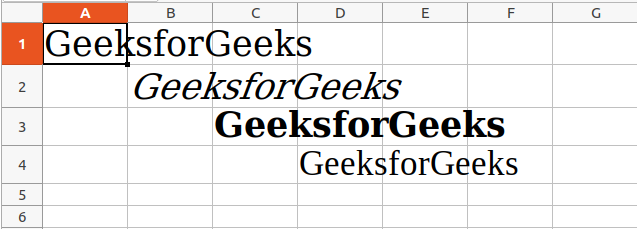Refer to the below article to get detailed information about adjusting rows and columns.

## Plotting Charts

Charts are composed of at least one series of one or more data points. Series themselves are comprised of references to cell ranges. For plotting the charts on an excel sheet, firstly, create chart objects of specific chart class( i.e BarChart, LineChart, etc.). After creating chart objects, insert data in it, and lastly, add that chart object in the sheet object.

Example 1:

## Python3

 `# import openpyxl module``import` `openpyxl`` ` `# import BarChart class from openpyxl.chart sub_module``from` `openpyxl.chart ``import` `BarChart, Reference`` ` `# Call a Workbook() function of openpyxl``# to create a new blank Workbook object``wb ``=` `openpyxl.Workbook()`` ` `# Get workbook active sheet``# from the active attribute.``sheet ``=` `wb.active`` ` `# write o to 9 in 1st column of the active sheet``for` `i ``in` `range``(``10``):``    ``sheet.append([i])`` ` `# create data for plotting``values ``=` `Reference(sheet, min_col``=``1``, min_row``=``1``,``                   ``max_col``=``1``, max_row``=``10``)`` ` `# Create object of BarChart class``chart ``=` `BarChart()`` ` `# adding data to the Bar chart object``chart.add_data(values)`` ` `# set the title of the chart``chart.title ``=` `" BAR-CHART "`` ` `# set the title of the x-axis``chart.x_axis.title ``=` `" X_AXIS "`` ` `# set the title of the y-axis``chart.y_axis.title ``=` `" Y_AXIS "`` ` `# add chart to the sheet``# the top-left corner of a chart``# is anchored to cell E2 .``sheet.add_chart(chart, ``"E2"``)`` ` `# save the file``wb.save(``"sample.xlsx"``)`

Output: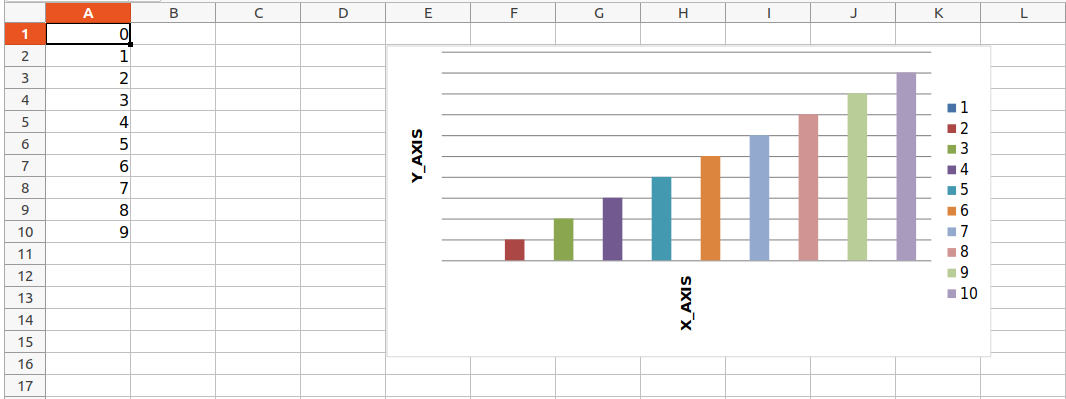Example 2:

## Python3

 `# import openpyxl module``import` `openpyxl`` ` `# import LineChart class from openpyxl.chart sub_module``from` `openpyxl.chart ``import` `LineChart, Reference`` ` `wb ``=` `openpyxl.Workbook()``sheet ``=` `wb.active`` ` `# write o to 9 in 1st column of the active sheet``for` `i ``in` `range``(``10``):``    ``sheet.append([i])`` ` `values ``=` `Reference(sheet, min_col``=``1``, min_row``=``1``,``                   ``max_col``=``1``, max_row``=``10``)`` ` `# Create object of LineChart class``chart ``=` `LineChart()`` ` `chart.add_data(values)`` ` `# set the title of the chart``chart.title ``=` `" LINE-CHART "`` ` `# set the title of the x-axis``chart.x_axis.title ``=` `" X-AXIS "`` ` `# set the title of the y-axis``chart.y_axis.title ``=` `" Y-AXIS "`` ` `# add chart to the sheet``# the top-left corner of a chart``# is anchored to cell E2 .``sheet.add_chart(chart, ``"E2"``)`` ` `# save the file``wb.save(``"sample.xlsx"``)`

Output: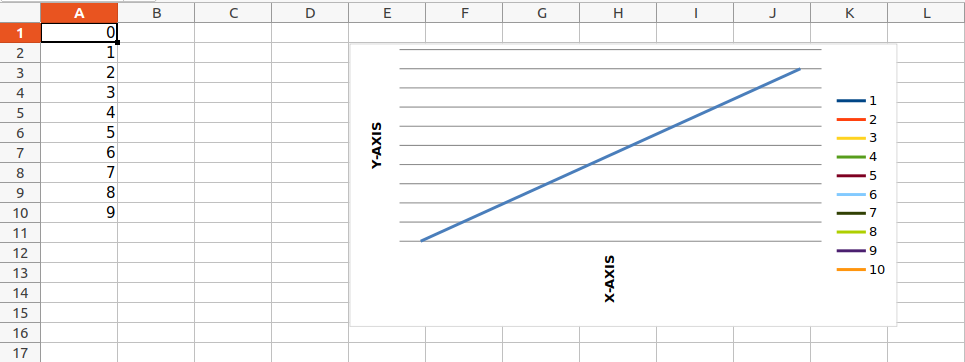Refer to the below articles to get detailed information about plotting in excel using Python.

For the purpose of importing images inside our worksheet, we would be using openpyxl.drawing.image.Image. The method is a wrapper over PIL.Image method found in PIL (pillow) library. Due to which it is necessary for the PIL (pillow) library to be installed in order to use this method.

Image Used:Example:

## Python3

 `import` `openpyxl ``from` `openpyxl.drawing.image ``import` `Image`` ` `wb ``=` `openpyxl.Workbook() `` ` `sheet ``=` `wb.active`` ` `# Adding a row of data to the worksheet (used to ``# distinguish previous excel data from the image) ``sheet.append([``10``, ``2010``, ``"Geeks"``, ``4``, ``"life"``]) `` ` `# A wrapper over PIL.Image, used to provide image ``# inclusion properties to openpyxl library ``img ``=` `Image(``"geek.jpg"``)`` ` `# Adding the image to the worksheet ``# (with attributes like position) ``sheet.add_image(img, ``'A2'``) `` ` `# Saving the workbook created``wb.save(``'sample.xlsx'``)`

Output: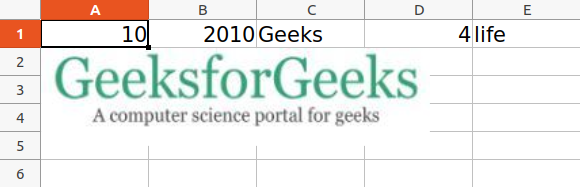Refer to the below article to get detailed information about adding images.

## Some More Functionality of Excel using Python

My Personal Notes arrow_drop_up